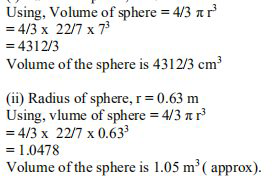# NCERT Solutions Class 9 Mathematics Solutions for Surface Areas and Volumes - Exercise 13.8 in Chapter 13 - Surface Areas and Volumes

Question 1 Surface Areas and Volumes - Exercise 13.8

Find the volume of a sphere whose radius is

(i) 7 cm (ii) 0.63 m

(Assume π=22/7)

The capacity of a sphere is measured by its volume. . Cubic units, such as m3, cm3, in3, and so on, are used to measure the volume of a sphere. The sphere is round and three-dimensional in shape. It has three axes that define its shape: the x-axis, y-axis, and z-axis.

(i) Radius of sphere, r = 7 cmRelated Questions

Lido

Courses

Teachers

Book a Demo with us

Syllabus

Maths
CBSE
Maths
ICSE
Science
CBSE

Science
ICSE
English
CBSE
English
ICSE
Coding

Terms & Policies

Selina Question Bank

Maths
Physics
Biology

Allied Question Bank

Chemistry
Connect with us on social media!